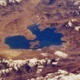# Unresolved Detail In Plotted Equations

Sometimes the calculator detects that an equation is too complicated to plot perfectly in a reasonable amount of time. When this happens, the equation is plotted at lower resolution.

267 out of 297 found this helpful
Have more questions? Submit a request

•Morgan Daugherty

sin(x^2)+sin(y^2)=0

•Infinite Planes

sin(x/y - y/x)=0

•Sean Budds

\tan \left(\operatorname{arcsinh}\left(1-x^{\operatorname{arccoth}\left(\frac{\csc \left(\operatorname{nPr}\left(x,5\right)\right)}{1\cdot y^{\left(\operatorname{arccsc}\left(y+1\right)\right)}}\right)}-y\right)\right)=\operatorname{arctanh}\left(\sin \left(x\left(y^{\left(x-\sin 5x\right)}\right)\right)\right)

...

•Sean Budds

\tan \left(\sin x^2\right)=\arctan \left(\sin y^2\right)

•Mateo Zaki

y=20cos(2/7x)tan(y!^x!)sin(1/3y)

•Mateo Zaki

It's a queso turkey surprise!

•Cldonkin
•Vigneshanand06

y^{-2}=\left|\left(3x\right)\right|^{3x}

-f\left|\left(xy\right)\right|=-\left(x^{\frac{2y}{x}\cdot -f}\right)

NOTE: Add a slider to f in the second one.

\frac{\sin xxxxyyyyy}{\frac{\sin x}{\sin y}}=y

\left(\sin \left(xy\left(\tan \left(x\right)\cdot \cos \left(y\right)\right)\right)\right)=y

\frac{\left(\sin \left(xy\left(\tan \left(x\right)\cdot \cos \left(y\right)\right)\right)\right)}{\sin \left(x^{\frac{x}{y}}\right)}=y

•Vigneshanand06

https://www.desmos.com/calculator/wm6djsyczg

play the slider to see movement

•Mrei6641

x^y=\cos \left(x\right)

if you zoom out, beware of lag(bad pc's)

•- Aylizior -

I saw a recurring pattern of basically sinx=siny here... so I took that and built a holy pattern of greatness:

$\tan \left(\frac{1x}{\cos \left(y\right)}\right)\le \tan \left(\frac{1y}{\cos \left(x\right)}\right)$

•Lawrence Tran
•Lawrence Tran

y=x!^{\sin \left(\frac{y}{\sqrt{\tan \left(x\right)}}\right)}

Is cool.

•Kacper Słomiński

x=sin(x^y) y=cos(y^x)

•Allendan001

\left|\operatorname{round}\left(y!\right)+\operatorname{floor}\left(x!\right)\right|=\operatorname{ceil}\left(xy!\right)

•Xinli Dai the Pi Guy

try y^a=sin(xb) or any other function

•Xinli Dai the Pi Guy
•Josh Sherman

8\cdot x^{\log 9^x}=\ \ln y just kills it

•Ping YuThe effect of this unresolved detailing issue made a red cross logo for me!

Copy and paste to help spread Red Cross!

•Jamestolliver

(p)cos((x^2)(sin(y^2)))=(q)cos((y^2)(sin(x^2)))

- p and q are sliders - press play and watch the lag

•Ahmed Shaikha

send to me your calculator breaking equations

look for graphs called crazy

Edited by Ahmed Shaikha
•Pedro Et Cetera

\sin \left(\cos \left(\tan \left(x^y\right)\right)\right)=\sin \left(\cos \left(\tan \left(y^x\right)\right)\right)

•Pedro Et Cetera

I compiled A bunch found on this thread and a few of my own, all of them are hidden and turn them on at your own risk. My computer could handle about 6 or 7 before it started lagging but not all computers are the same

https://www.desmos.com/calculator/gktoiurlai

•Grhumphrey20
Edited by Grhumphrey20
•Grhumphrey20

$\left(\log _x\left(\tan \left(y^x\right)\right)\right)^{\frac{\operatorname{sign}\left(\sin \left(x^{\sin \left(y\right)}\right)\right)}{\sqrt[\cos x]{\operatorname{sign}\left(x^{y\sin \left(x\right)}\right)}}}=0.5$

•Xklein 2021

Square root of xy = x^y is one

•Xinli Dai the Pi Guy

I just broke desmos: |sinx^y|=|y^sinx|

Edited by Xinli Dai the Pi Guy
•Sjii1829

i think i broke desmos even more https://www.desmos.com/calculator/4oyilidgdu

•Ahmed Shaikha

i don't need repeats of those already given. the graph is already lagging like crasy

•Liueri

\left(\sin x\right)^{\left(\cos y\right)}=\left(\cos x\right)^{\left(\sin y\right)} would make a nice wallpaper...